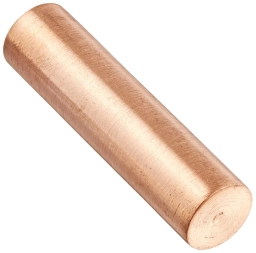# Calculate 82549

The cylinder has a shell surface of 88 square cm and a volume of 176 cubic cm. Calculate the radius, height, and surface area of the given solid

r =  4 cm
h =  3.5014 cm
S2 =  188.531 cm2

### Step-by-step explanation:Did you find an error or inaccuracy? Feel free to write us. Thank you!

Tips for related online calculators
Need help calculating sum, simplifying, or multiplying fractions? Try our fraction calculator.
Do you want to convert length units?